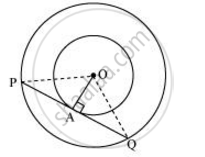# Two concentric circles are of radii 5 cm and 3 cm. Find the length of the chord of the larger circle which touches the smaller circle - Mathematics

Two concentric circles are of radii 5 cm and 3 cm. Find the length of the chord of the larger circle which touches the smaller circle

#### SolutionLet the two concentric circles be centered at point O. And let PQ be the chord of the larger circle which touches the smaller circle at point A. Therefore, PQ is tangent to the smaller circle.

OA ⊥ PQ (As OA is the radius of the circle)

Applying Pythagoras theorem in ΔOAP, we obtain

OA+ AP= OP2

3+ AP= 52

9 + AP= 25

AP= 16

AP = 4

In ΔOPQ,

Since OA ⊥ PQ,

AP = AQ (Perpendicular from the center of the circle bisects the chord)

∴ PQ = 2AP = 2 × 4 = 8

Therefore, the length of the chord of the larger circle is 8 cm.

Concept: Number of Tangents from a Point on a Circle
Is there an error in this question or solution?

#### APPEARS IN

NCERT Class 10 Maths
Chapter 10 Circles
Exercise 10.2 | Q 7 | Page 214